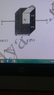• vijayramakrishnan
In summary, the ratio of capacitance C2 to C1 in a parallel plate capacitor with two dielectrics of different relative permittivities (ε1=2 and ε2=4) is not affected by connecting 1 and 4 in parallel and 2 and 3 in parallel and then connecting them in series. This is because the electric field between 1 and 2 is not the same as the electric field between 3 and 4.

## Homework Statement

A parallel plate capacitor having plates of area S and plate separation d, has capacitance C1 in air. When two dielectrics of different relative permittivities ( ε1=2 and ε2=4) are introduced between the two plates as shown in the figure, the capacitance becomes C2. the ratio C2/C1 is

C= ε0A/d[/B]

## The Attempt at a Solution

if we name the components 1,2,3,4 in clockwise manner,in the solution 1 and 2 are taken in series and 3 and 4 are taken in series and they both are connected parallel,can we also take 1 and 4 in parallel and 2 and 3 in parallel and they both are connected in series?in that case answer comes out to be 12/5 where have i gone wrong?
[/B]

#### Attachments

•DSC_0473.JPG
14.8 KB · Views: 394
Last edited:
vijayramakrishnan said:
can we also take 1 and 4 in parallel and 2 and 3 in parallel and they both are connected in series?
No. Electric field between 1 and 2 (left half) is not same as the electric field between 3 and 4(left half).

cnh1995 said:
No. Electric field between 1 and 2 (left half) is not same as the electric field between 3 and 4(left half).
sir 2 is not left half,1234 in clockwise direction

vijayramakrishnan said:
sir 2 is not left half,1234 in clockwise direction
sir,do you mean to say that the potential difference between positive terminal and interface between 1 and 2 is not same as potential difference between positive terminal and interface between 3 and 4.

cnh1995 said:
No. Electric field between 1 and 2 (left half) is not same as the electric field between 3 and 4(left half).
sir,do you mean to say that the potential difference between positive terminal and interface between 1 and 2 is not same as potential difference between positive terminal and interface between 3 and 4.